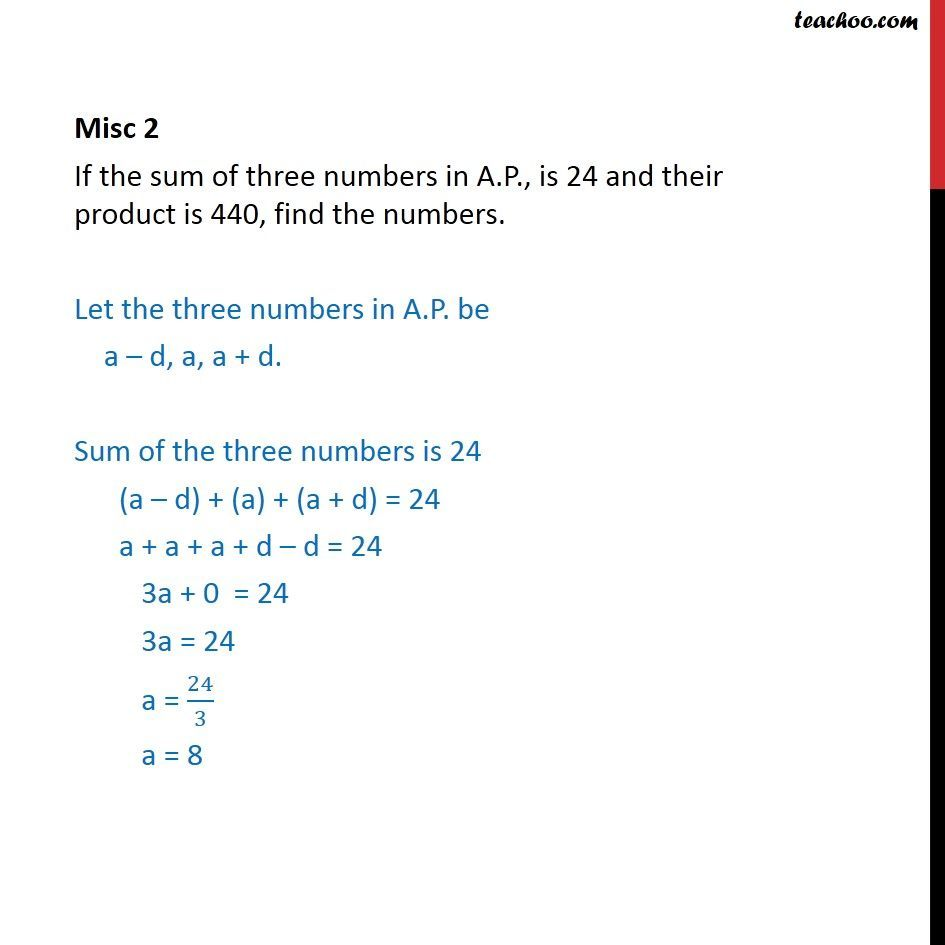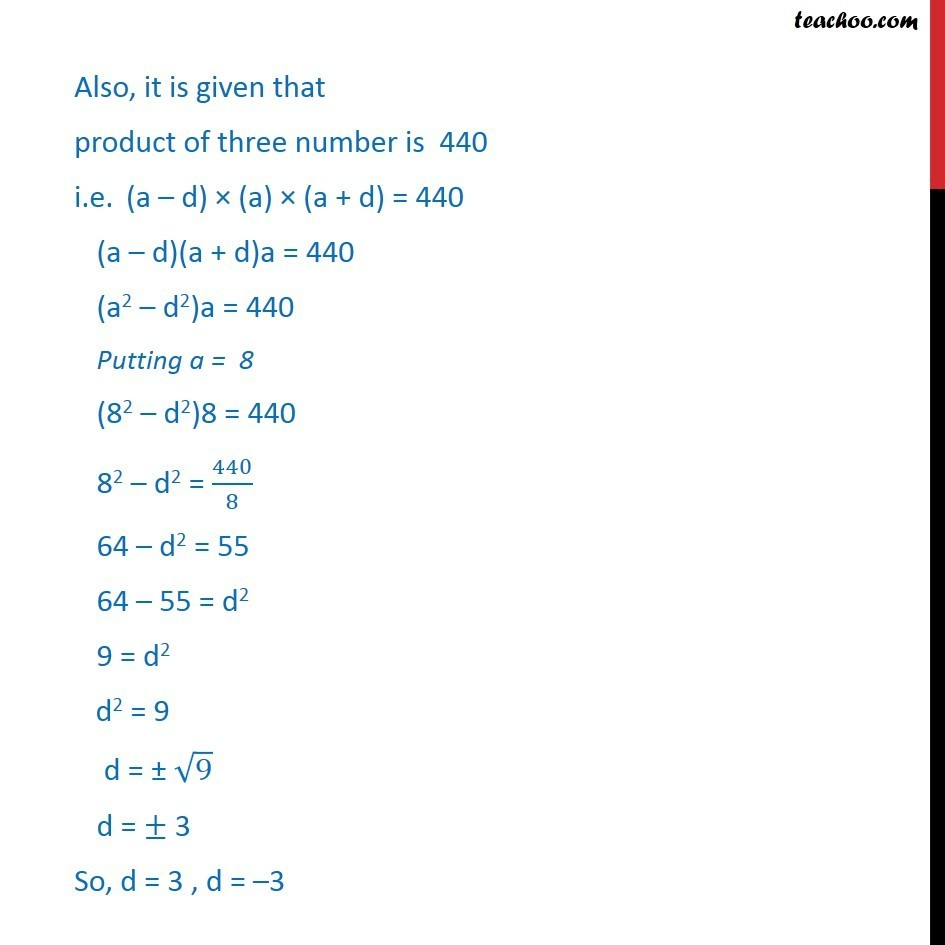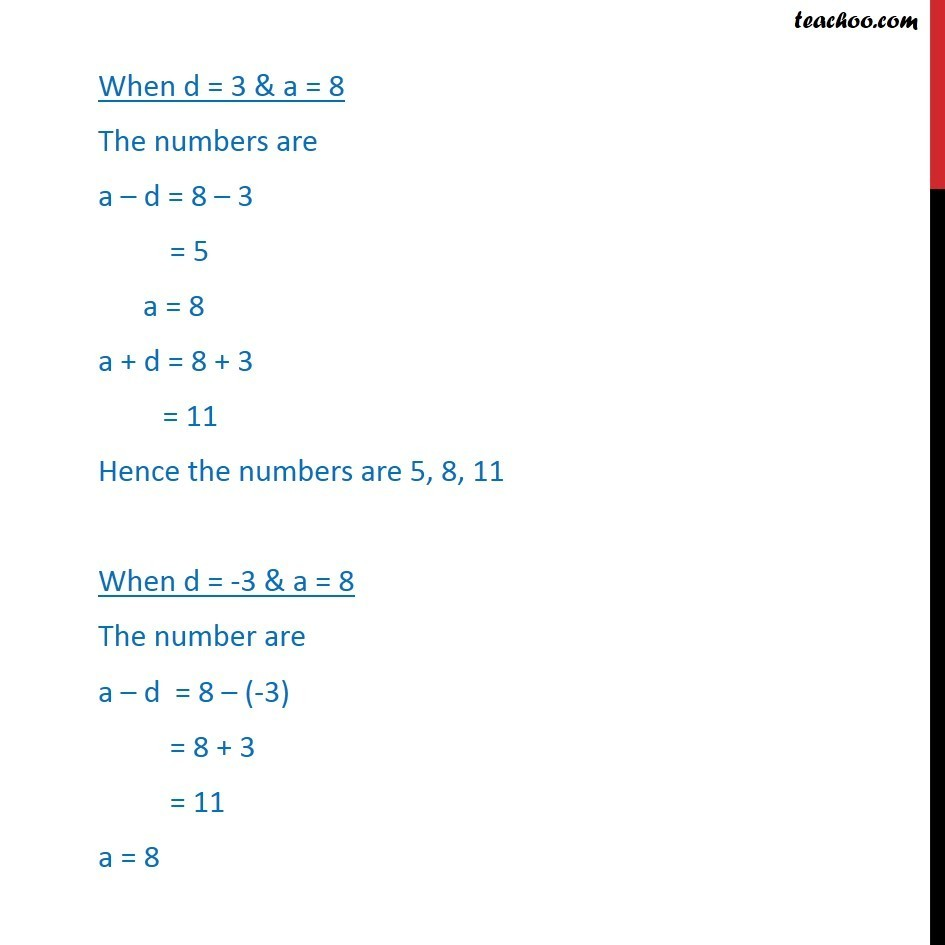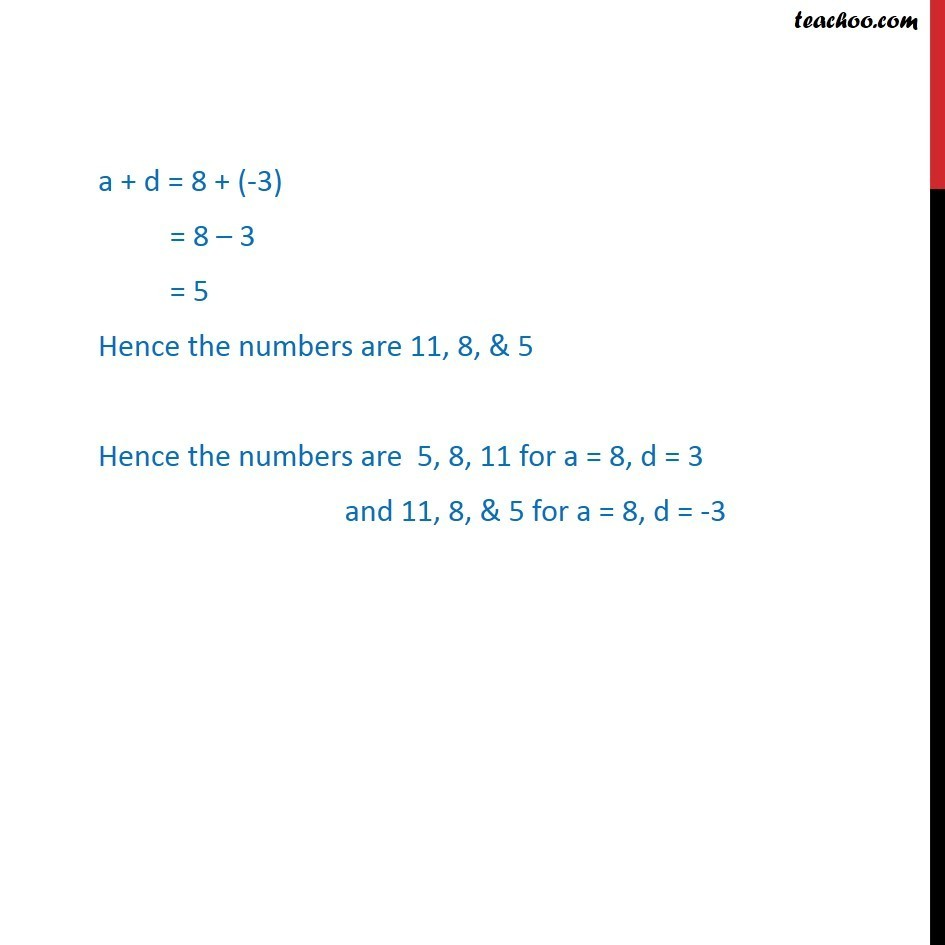Miscellaneous

Chapter 8 Class 11 Sequences and Series
Serial order wiseLearn in your speed, with individual attention - Teachoo Maths 1-on-1 Class

### Transcript

Question 2 If the sum of three numbers in A.P., is 24 and their product is 440, find the numbers. Let the three numbers in A.P. be a d, a, a + d. Sum of the three numbers is 24 (a d) + (a) + (a + d) = 24 a + a + a + d d = 24 3a + 0 = 24 3a = 24 a = 24/3 a = 8 Also, it is given that product of three number is 440 i.e. (a d) (a) (a + d) = 440 (a d)(a + d)a = 440 (a2 d2)a = 440 Putting a = 8 (82 d2)8 = 440 82 d2 = 440/8 64 d2 = 55 64 55 = d2 9 = d2 d2 = 9 d = 9 d = 3 So, d = 3 , d = 3 When d = 3 & a = 8 The numbers are a d = 8 3 = 5 a = 8 a + d = 8 + 3 = 11 Hence the numbers are 5, 8, 11 When d = -3 & a = 8 The number are a d = 8 (-3) = 8 + 3 = 11 a = 8 a + d = 8 + (-3) = 8 3 = 5 Hence the numbers are 11, 8, & 5 Hence the numbers are 5, 8, 11 for a = 8, d = 3 and 11, 8, & 5 for a = 8, d = -3